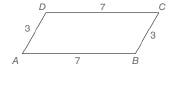Chapter 4.2, Problem 1EElementary Geometry For College St...

7th Edition
Alexander + 2 others
ISBN: 9781337614085

Solutions

Chapter
SectionElementary Geometry For College St...

7th Edition
Alexander + 2 others
ISBN: 9781337614085
Textbook Problem

Note: Exercises preceded by an asterisk are of a more challenging nature.a) As shown, must quadrilateral ABCD be a parallelogram? b ) Given the lengths of the sides as shown, is the measure of ∠ A unique?To determine

a)

To check:

Whether the given quadrilateral is a parallelogram.

Explanation

Given,

AB=7 units

BC=3 units

CD=7 units

DA=3 units and

ABCD is a quadrilateral.

Draw the diagonal AC¯ as exactly one line passes through two points.

Now, we have two triangles ABC and CDA, such that

AC<

To determine

b)

Whether the measure of A is unique for the given lengths of the sides.

Still sussing out bartleby?

Check out a sample textbook solution.

See a sample solution

The Solution to Your Study Problems

Bartleby provides explanations to thousands of textbook problems written by our experts, many with advanced degrees!

Get Started

Draw, in standard position, the angle whose measure is given. 18. 150

Single Variable Calculus: Early Transcendentals, Volume I

Solve

Mathematical Applications for the Management, Life, and Social Sciences

Convert the expressions in Exercises 6584 to power form. 3x453x+43xx

Finite Mathematics and Applied Calculus (MindTap Course List)

Let h be the function defined by h(x) = x3 x2 + x + 1. Find h(5), h(0), h(a), and h(a).

Applied Calculus for the Managerial, Life, and Social Sciences: A Brief Approach

True or False: All the hypotheses for the Mean Value Theorem hold for f(x) = 1 − |x| on [−1, 2].

Study Guide for Stewart's Single Variable Calculus: Early Transcendentals, 8th